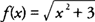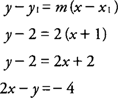## Tangent and Normal Lines

The derivative of a function has many applications to problems in calculus. It may be used in curve sketching; solving maximum and minimum problems; solving distance; velocity, and acceleration problems; solving related rate problems; and approximating function values.

The derivative of a function at a point is the slope of the tangent line at this point. The normal line is defined as the line that is perpendicular to the tangent line at the point of tangency. Because the slopes of perpendicular lines (neither of which is vertical) are negative reciprocals of one another, the slope of the normal line to the graph of f(x) is −1/ f′(x).

Example 1: Find the equation of the tangent line to the graph ofat the point (−1,2).At the point (−1,2), f′(−1)=−½ and the equation of the line isExample 2: Find the equation of the normal line to the graph ofat the point (−1, 2).

From Example 1, you find that f′(−1)=−½ and the slope of the normal line is −1/ f′(−1) = 2; hence, the equation of the normal line at the point (−1,2) is